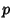## Fundamental Continuity Theorem

Given two Polynomials of the same order in one variable where the firstCoefficients (but not the first) are 0 and the Coefficients of the second approach the corresponding Coefficients of the first as limits, then the second Polynomial will have exactlyroots that increase indefinitely. Furthermore, exactlyRoots of the second will approach each Root of multiplicityof the first as a limit.

References

Coolidge, J. L. A Treatise on Algebraic Plane Curves. New York: Dover, p. 4, 1959.